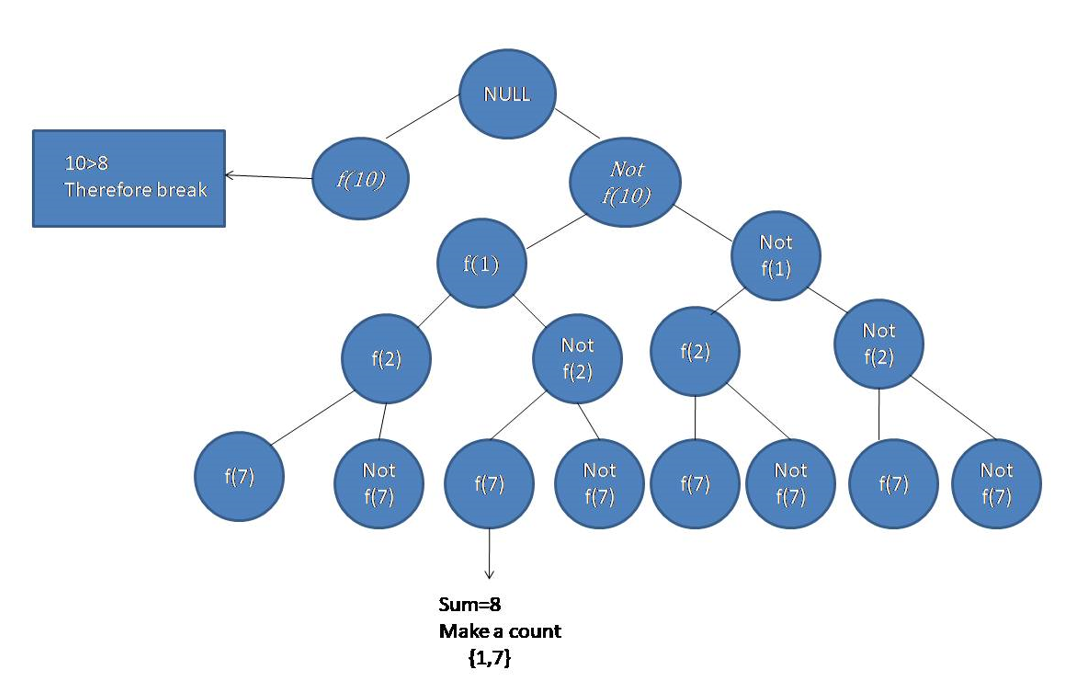# Combinational sum problem

Combinational sum problem: Here, we are going to learn to make some combination of the numbers whose sum equals to a given number using backtracking.
Submitted by Souvik Saha, on February 07, 2020

Description:

This is a standard interview problem to make some combination of the numbers whose sum equals to a given number using backtracking.

Problem statement:

Given a set of positive numbers and a number, your task is to find out the combinations of the numbers from the set whose summation equals to the given number.

```    Input:
Test case T

T no. of N values and corresponding N positive numbers and the Number.

E.g.
3
6
4 1 3 2 4 5
8

7
4 5 2 5 1 3 4
10

7
10 1 2 7 6 1 5
8

Constrains:
1 <= T <= 500
1 <= N <= 20
1 <= A[i] <= 9
1 <= Number<= 50

Output:
Print all the combination which summation equals to the given number.
```

Example

```    Input:
N = 7

Set[] = 10 1 2 7 6 1 5
Number = 8

Output:
1 1 6
1 2 5
1 7
2 6
```

Explanation with example

Let there is a set S of positive numbers N and a positive number.

Making some combinations in such a way that the summation of that combination results that given number is a problem of combination and we will solve this problem using a backtracking approach.

```    Let,
f(i) = function to insert the ith number into the combinational subset.
```

In this case, we will consider two cases to solve the problem,

1. We will consider ith element into the part of our combination subset. (f(i))
2. We will not consider ith element into the part of our combination subset.(not f(i))

And every time we will check the current sum with the number. Each of the time we will count the number of occurrence and the also the combinations.

```    Let,
f(i) = function to insert the ith number into the combinational subset.
```

For the input:

```    S[] = {10, 1, 2, 7, 6, 1, 5}
Number = 8
```Here in this case we will discard that edges which have a current sum greater than the given number and make a count to those numbers which are equal to the given number.

C++ implementation:

```#include <bits/stdc++.h>
using namespace std;

void combination(int* arr, int n, int num, int pos, int curr_sum, vector<vector<int> >& v, vector<int> vi, set<vector<int> >& s)
{
if (num < curr_sum)
return;
if (num == curr_sum && s.find(vi) == s.end()) {
s.insert(vi);
v.push_back(vi);
return;
}
//go for the next elements for combination
for (int i = pos; i < n; i++) {
if (curr_sum + arr[i] <= num) {
vi.push_back(arr[i]);
combination(arr, n, num, i + 1, curr_sum + arr[i], v, vi, s);
vi.pop_back();
}
}
}

//print the vector
void print(vector<vector<int> > v)
{
for (int i = 0; i < v.size(); i++) {
for (int j = 0; j < v[i].size(); j++) {
cout << v[i][j] << " ";
}
cout << endl;
}
}

void combinational_sum(int* arr, int n, int num)
{
vector<vector<int> > v;
vector<int> vi;
int pos = 0;
int curr_sum = 0;
set<vector<int> > s;
combination(arr, n, num, pos, curr_sum, v, vi, s);
print(v);
}

int main()
{
int t;

cout << "Test Case : ";
cin >> t;

while (t--) {
int n, num;

cout << "Enter the value of N : ";
cin >> n;

int arr[n];
cout << "Enter the values : ";
for (int i = 0; i < n; i++) {
cin >> arr[i];
}

sort(arr, arr + n);

cout << "Enter the number : ";
cin >> num;

combinational_sum(arr, n, num);
}
return 0;
}
```

Output

```Test Case : 3
Enter the value of N : 6
Enter the values : 4 1 3 2 4 5
Enter the number : 8
1 2 5
1 3 4
3 5
4 4
Enter the value of N : 7
Enter the values : 4 5 2 5 1 3 4
Enter the number : 10
1 2 3 4
1 4 5
2 3 5
2 4 4
5 5
Enter the value of N : 7
Enter the values : 10 1 2 7 6 1 5
Enter the number : 8
1 1 6
1 2 5
1 7
2 6
```

What's New (MCQs)

Top Interview Coding Problems/Challenges!

Languages: » C » C++ » C++ STL » Java » Data Structure » C#.Net » Android » Kotlin » SQL
Web Technologies: » PHP » Python » JavaScript » CSS » Ajax » Node.js » Web programming/HTML
Solved programs: » C » C++ » DS » Java » C#
Aptitude que. & ans.: » C » C++ » Java » DBMS
Interview que. & ans.: » C » Embedded C » Java » SEO » HR
CS Subjects: » CS Basics » O.S. » Networks » DBMS » Embedded Systems » Cloud Computing
» Machine learning » CS Organizations » Linux » DOS
More: » Articles » Puzzles » News/Updates

© https://www.includehelp.com some rights reserved.